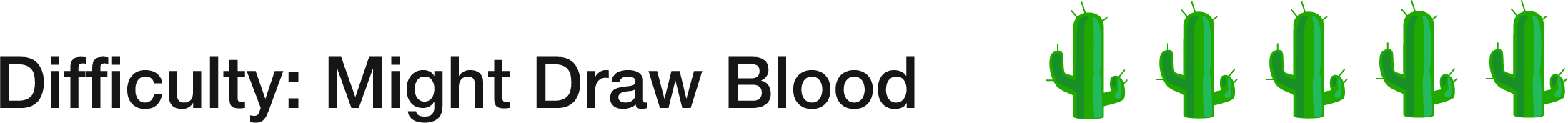# 11 of 100: 999 Sentences

Logic Level 3

Suppose the alternating pattern below continues for 998 sentences before ending with one unique sentence.

1) The next sentence is $\color{#3D99F6}\text{true}\color{#333333}.$

2) The next sentence is $\color{#D61F06}\text{false}\color{#333333}.$

3) The next sentence is $\color{#3D99F6}\text{true}\color{#333333}.$

4) The next sentence is $\color{#D61F06}\text{false}\color{#333333}.$
...
997) The next sentence is $\color{#3D99F6}\text{true}\color{#333333}.$

998) The next sentence is $\color{#D61F06}\text{false}\color{#333333}.$

999) $Y\geq5$

I'm thinking of a number, $Y.$ If sentence 1 is true, what do you know about $Y$?Be systematic! In our opinion, this is the hardest problem so far, especially if you play around with generalizing it to more complex patterns of "The next sentence is $\color{#3D99F6}\text{true}\color{#333333}/ \color{#D61F06}\text{false}\color{#333333}$" than the alternating pattern above. We hope you enjoy it! :)

×